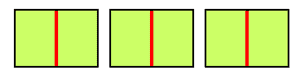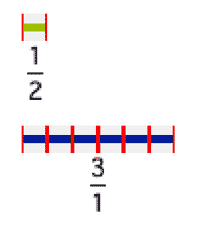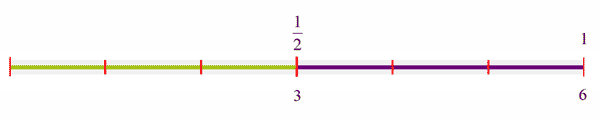# 3 ÷ ½

Using visual models can help students better understand mathematics problems.

Often, using visual models can help students better understand mathematics problems. How can the following drawings be interpreted as showing the quotient for 3 ÷ ½? What real-world problem might go with each of the three diagrams?

a)b)c)